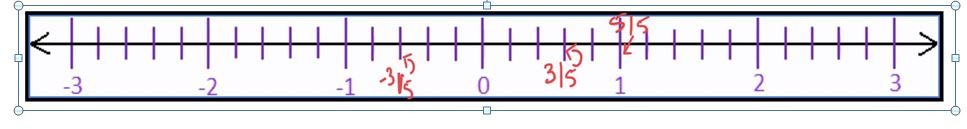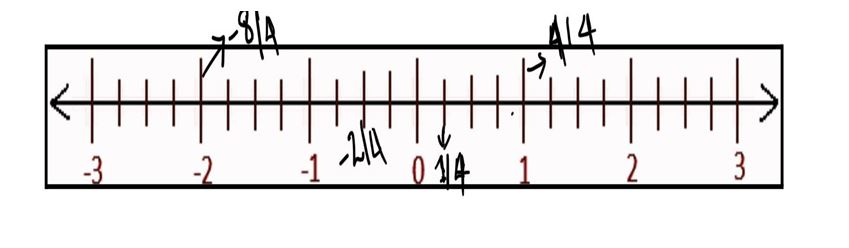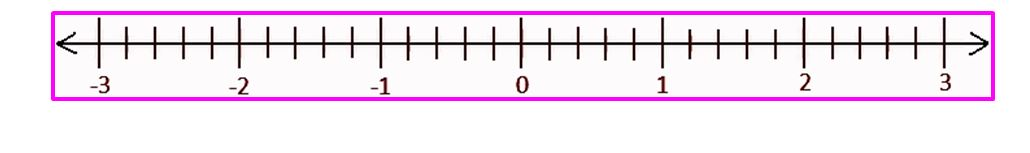###### Latest Topics## Fractions on a Number Line

Representing fractions on a number line illustrates the intervals between two integers. This contributes to the development of the fundamental concept of fractional number formation.

## 1. On a number line, represent the fractions 3/5, 5/5, and -3/5.

Check the denominator of each given fraction. You notice that it is five. Now, it is time to split the space between any two consecutive integer pairs into five equal pieces on the number line.

Each component acquired in this manner will represent the fraction 1/5, and the resulting number line will have the form:For 3/5; move three parts on the right of zero.

For 5/5; move five parts on the right of zero.

For -3/5; move three parts on the left of zero.

2. On a number line, represent the fractions 1/4, 4/4, -2/4, and -8/4.

Check the denominator of each given fraction. You notice that it is four. Now, it is time to split the space between any two consecutive integer pairs into four equal pieces on the number line. Each component acquired in this manner will represent the fraction 1/4, and the resulting number line will have the form:For 1/4; move one part on the right of zero.

For 4/4; move four parts on the right of zero.

For -2/5; move two parts on the left of zero.

For -8/4; move eight parts on the left of zero.

Number Line: The number line has a straight line with equal numbers along its length.

A number line has equal segments or intervals between each number. Hence numbers are placed at an equal distance or parts from each other.

Number lines can be extended in any direction indefinitely. You can extend it on the left and right sides infinitely. We usually represent the number line with numbers on it on a horizontal line.

Moving from left to right:As we move left to right along the number line, numbers (magnitude) increases. For example, consider you are in zeroth position on a number line. As you move towards the right, 1 will appear, then 2, then 3, then 4, and so on. Thus, the number increases as we move from left to right. See the image above.

Moving from right to left:

On the other hand, the numbers decrease moving from right to left. Suppose you are on number three on the number line. If you move towards the left, the number decreases. That is, it would become 2, then 1, zero, and the negative number line start, that is -1. Thus the number decreases as we move from right to left. Check the image above.

FAQs

How I do divide the number line?

Ans. To divide the number line into equal parts, check what is the denominator first. Split the number line into two equal segments or parts based on the denominator. For example, if the fraction is 7/5. Initially, divide the number line into 5 equal parts. Then move to 7 on the right side, starting from zero.